# IBPS RRB Officer Scale Exam 2018: Complete Practice Set of Reasoning Ability

IBPS has released the notification of for the next Common Recruitment Process for Regional Rural Banks (RRBs) (CRP RRBs VII) for recruitment of Officers (Scale-I, II & III) and Office Assistant (Multipurpose). Here is the practice set of Reasoning Ability for the IBPS RRB officer scale exams.

Created On: Jun 11, 2018 13:26 ISTIBPS RRB Exam: Practice Set Reasoning

Reasoning questions with answers for IBPS RRB 2018 exams are available here. You can also expect similar questions in other bank recruitment exams (i.e., IBPS PO, SBI PO, SBI Clerk, NABARD etc) besides IBPS RRB.

IBPS RRB 2018 Recruitment notification has been recently released. It contains complete detail about syllabus, exam pattern, vacancies etc. You can get all the important details about IBPS RRB 2018 Recruitment Notification from the link given below:

IBPS RRB 2018: Important Dates, Exam Pattern, Syllabus, Eligibility Criteria and More

As per latest exam pattern, 40 questions in the Reasoning Section will be asked. So, here we have provided 40 questions with answers (as per latest trend of IBPS RRB). Similar questions are expected to be asked in preliminary exams [Officers (Scale-I, II & III) and Office Assistant (Multipurpose)] of IBPS RRB 2018.

Directions (1-5): Study the following digit-letter-symbol sequence carefully and answer the questions given below:

S * V G L 2 \$ D = H # 9 N % B < K 5 & A Y ? U E + Z @ 7 F 6

1. Which of the following is sixth to the left of eighteenth element from the left?

a)      %

b)      C

c)      5

d)      9

e)      None of these

Answer (d)

2.      If the above sequence is written in reverse order then which of the following will be sixth to the right of sixteenth element from the right end?

a)      H

b)      A

c)      K

d)      U

e)      None of these

Answer (a)

3.      If the first fifteen elements in the above sequence are written in reverse order, then which of the following will be twenty-fourth from the right end?

a)      2

b)      \$

c)      =

d)      #

e)      None of these

Answer (d)

4.      What should come in place of question mark in the following on the basis of above sequence?

* S G : F 6 @ L G \$ : Z @ E D \$ H : ?

a)      # H N

b)      U E Y

c)      U ? +

d)      U E ?

e)      None of these

Answer (b) Explanation: * S G : F 6 @ L G \$ : Z @ E D \$ H : U E Y.

5.      How many such numbers are there in the above sequence, each of which is immediately preceded by a consonant and immediately followed by a symbol?

a)      Nil

b)      One

c)      Three

d)      Four

e)      None of these

Answer (e) Explanation:  L 2 \$, K 1 &.

IBPS RRB Officer Scale Exam 2017: Complete Practice Set of Quantitative ‎Aptitude‎

Directions (6 - 10): Study the following information and answer the questions given below:

Eight friends – F1, F2, F3, F4, F5, F6, F7 and F8 – are sitting around a circular table not necessarily in the same order. Three of them are facing outward while five are facing towards the centre. There are equal number of males and females in the group.

F3 is facing the centre. F5 is sitting third to the right of F3. F6 is sitting third to the left of F5. Three persons are sitting between F6 and F2. The immediate neighbors of F2 are females. F7 is sitting third to the right of F6. F4 is sitting third to the right of F1. F1 is not an immediate neighbour of F5. The immediate neighbors of F5 are males and are facing the centre. The immediate neighbors of F4 are females and face outside. The one sitting third to the left of F2 is a male. No female is an immediate neighbour of F7.

6. Who is sitting second to the right of F5?

a)      F3

b)      F2

c)       F7

d)      F8

e)      None of these

Answer (c)

7.    How many persons are sitting between F8 and F3 when counted from the left side of F8?

a)      One

b)      Two

c)       Three

d)      Four

e)      More than four

Answer (b)

8. Which of the following statements is true regarding F8?

a)      The one who is second to the right of F8 is a female.

b)      F8 is facing the centre.

c)       F8 is a male.

d)      The immediate neighbours of F8 are facing outside.

e)      None is true

Answer (a)

9. What is F4’s position with respect to F7?

a)      Third to the left

b)      Third to the right

c)       Second to the left

d)      Second to the right

e)      None of these

Answer (b)

10. If all the friends are asked to sit in an alphabetical order starting from F1 in an anti clockwise direction, the positions of how many will remain unchanged (excluding F1)?

a)      Four

b)      Three

c)       Two

d)      One

e)      None

Answer (d) Explanation:

Male-F1F2F3F4, Female-F5F6F7F8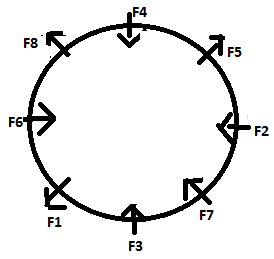Directions (11-15): Study the following information carefully and answer the given questions.

Eight friends F1, F2, F3, F4, F5, F6, F7 and F8 are sitting in a straight line facing north. F8 is on the immediate right of F4, who is not at the end of the row. F2 is on the immediate left of F5 but F2 is neither a neighbour of F1 nor sits at the ends of the row. F3 and F5 are not adjacent to each other. There are three persons between F2 and F8. F1 is adjacent to F6, who is on the immediate left of F3. F7 is second to the left of F8 and is on the immediate right of F5.

11. Which of the following statements is true?

a)      F2 is in the middle of the row.

b)      F8 is at the right end of the row.

c)       F1 is second to the left of F2.

d)      All are true

e)      None of these

Answer (b)

12. Which of the following pairs sit at the ends of the row?

a)      F2F7

b)      F2F7

c)       F1F8

d)      F3F2

e)      None of these

Answer (c)

13. If F3 faces south then how many persons will be sitting to the right of F3?

a)      One

b)      Two

c)       Three

d)      Four

e)      None of these

Answer (b)

14. If F1 related to F8 and F6 is related to F4 in a certain way, then following the same way, F3 is related to which of the following?

a)      F8

b)      F2

c)       F7

d)      F5

e)      None of these

Answer (c)

15. Which of the following persons sits second to the right of F2?

a)      F4

b)      F1

c)       F3

d)      F7

e)      F8

Answer (d) Explanation: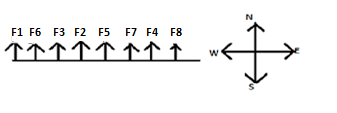Why you should read 'The Hindu' while preparing for Bank Exams

Directions (16-20): In each of the questions below are given four statements followed by three conclusions numbered I, II & III. You have to take the given statements to be true even if they seem to be at variance with commonly known facts. Read all the conclusions and then decide which of the given conclusions logically follows from the given statements disregarding commonly known facts.

16. Statements: All rectangles are squares. All squares are triangles. Some triangles are trapeziums. Some trapeziums are cones.

Conclusions:

I. Some triangles are rectangles.

II. Some cones are triangles.

III. Some trapeziums are squares.

a)      Only I follows

b)      Only I & II follow

c)       Only I & III follow

d)      All I, II & III follow

e)      None of these

Answer (a) Explanation: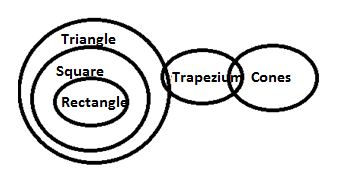17. Statements: Some visions are goals. Some goals are aims. Some goals are objectives. No objective is idea.

Conclusions:

I. Some aims are objectives.

II. No aims are objectives.

III. No idea is goal.

a)      None follows

b)      Only I follows

c)       Only II follows

d)      Only III follows

e)      Only either I or II follows

Answer (e) Explanation: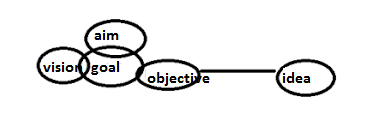18. Statements: Some days are weeks. Some weeks are months. All months are years.

All years are decades.

Conclusions:

I. All months are decades.

II. Some weeks are years.

III. Some weeks are decades.

a)      All follow

b)      Only I follows

c)       Only II & III follow

d)      Only I & II follow

e)      None of these

Answer (a) Explanation: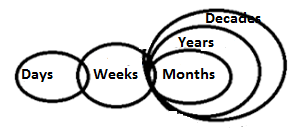19. Statements: Some cities are towns. Some towns are villages. No village is lanes. All lanes are colonies.

Conclusions:

I. No village is colony.

II. Some towns are lanes.

III. Some colonies are lanes.

a)      Only I follows

b)      Only III follows

c)       Only II & III follow

d)      None follows

e)      None of these

Answer (b) Explanation: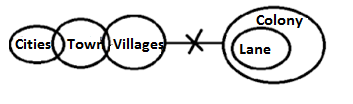20. Statements: Some shows are plays. No play is theatre. All theatres are movies.

Some movies are dramas.

Conclusions

I. No play is movie.

II. Some shows are dramas.

III. Some theatres are dramas.

a)      Only I follows

b)      Only II follows

c)       Only III follows

d)      None follows

e)      None of these

Answer (d) Explanation: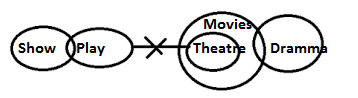21. Jatin starts at point T, walks straight to point U which is 4 ft away. He turns left at 90° and walks to W which is 4 ft away, turns 90° right and goes 3 ft to P, turns 90° right and walks 1 ft to Q, turns left at 90° and goes to V , which is 1 ft away and once again turns 90° right and goes to R, 3 ft away. What is the distance between T and R?

a)      4 ft

b)      5 ft

c)       7 ft

d)      8 ft

e)      None of these

Answer (d) Explanation:

The starting point T and ending point R lie on the same line. The distance between T and R is 4 + 3 + 1 = 8feet.

22. Ranjan starts walking from his house to go to the nearby park. He travels 5m to the East, then travels 10m to the left and then travels 6m to the right. He further travels 2m North and finally travels 2m East to reach the park. How far is the park from his house?

a)      20m

b)      40m

c)       15m

d)      25m

e)      None of these

Answer (c) Explanation:

The shortest distance can be found out by using Pythagoras theorem = √ (122 + 132) = 15meters.

Examine the following relationship among members of a family of six persons - A, B, C, D, E and F.

• The number of males equals that of females.
• A and E are sons of F.
• D is the mother of two, one boy and one girl.
• B is the son of A.
• There is one married couple in the family at present.

23.Which one of the following inferences can be drawn from the above?

a)      A, B and C are all females.

b)      A is the husband of D.

c)       D is the granddaughter of F

d)      E and F are children of D.

e)      None of these

Answer (b) Explanation: It is clear that there are three males and three females in the family. Clearly A, E and B are males. So, C, D, and F are females. Also given that D is the mother of one boy and one girl. So, A and D form a couple having two children, namely B and C. Hence A is the husband of D.

24. Kavita starts from her home to go towards her office. She moves 2 km and turns right and travels 800m to reach a temple on the way. At the temple she turns left and moves 2 km and reaches her office. If Kavita is now travelling in East direction, in what direction is Kavita’s office with respect to her home?

a)      South

b)      South- East

c)      East

d)      South – West

e)      None of the above

Explanation: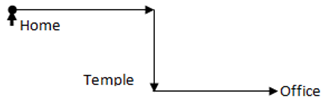Directions (25-29): Read the information / statement given in such question carefully and answer the questions.

25. Which of the following expressions will be true if the expression ‘M≤ J = L > F’ is definitely true?

a)      L ≥ M

b)      G = F

c)       M ≥ F

d)      F ≥ J

e)      None is true

Answer (a) Explanation:  L ≥ M, Will definitely hold true.

26. Which of the following expressions will not be true if the expression ‘X ≤ K < M = S’ is definitely true?

a)      S ≥ X

b)      X < M

c)       S > K

d)      X > S

e)      None of these

Answer (a) Explanation:  X ≤ K < M = S

S ≥ X, Will not be true

27. In which of the following expressions does the expression ‘C < E’ hold true?

a)      C > M < X ≤ E

b)      E > X ≤ M ≤ C

c)       E ≥ M > X ≥ C

d)      E > M = X < C

e)      E ≥ X > M ≤ C

Answer (c) Explanation:  C < E

E ≥ Y > X ≥ C

28.   In which of the following expressions will the expression ‘P < Q’ be definitely true?

a)      P ≥ A > N = Q

b)      P= A < N > Q

c)       P < A ≤ N ≤ Q

d)      Q ≥ A = B < P

e)      None of these

Answer (c) Explanation: P < Q, Will be true

In P < A ≤ N ≤ Q

29.   In which of the following expressions does the expression ‘Z > R’ does not hold true?

a)      R < B ≤ C = Z

b)      Z ≥ B > C ≥ R

c)       R ≤ B = C < Z

d)      Z ≥ C ≥ B > R

e)      R > B ≤ C = Z

Answer (e) Explanation: D > A, Does not hold true in expression (5): R > B ≤ C = Z

Examine the following relationship among members of a family of six persons - A, B, C, D, E and F.

• The number of males equals that of females.
• A and E are sons of F.
• D is the mother of two, one boy and one girl.
• B is the son of A.
• There is one married couple in the family at present.

30. Himanshu walked towards north in the direction of McDonalds. When McDonalds was 100 meters away from him, he turned left to pick Ravi who was 40 meters away in that direction. Both of them then walked towards the west for 100 meters and then turned right and walked further 100 meters. How many meters they are away from McDonalds now?

a)      0

b)      100

c)      40

d)      140

e)      None of the above

Answer: d)

Explanation: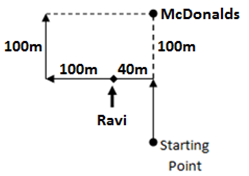Direction (31– 37): In each of the questions below consists of a question and two statements numbered I and II given below it. You have to decide whether the data provided in the statements are sufficient to answer the question. Read both the statements and give answer:

a)      If the data in statement I alone are sufficient to answer the question, while the data in statement II alone are not sufficient to answer the question;

b)      If the data in statement II alone are sufficient to answer the question, while the data in statement I alone are not sufficient to answer the question;

c)      If the data either in statement I alone or in statement II alone are sufficient to answer the         question;

d)      If the data given in both statements I and II together are not sufficient to answer the question; and

e)      If the data in both statements I and II together are necessary to answer the question.

31. Five students A, B, C, D and E are sitting in a row. Who is in the middle?

I.    Between A and B is C.

II.   C is to the right of A

Answer: d)

Explanation:          _ACB_, ACB _ _, _ _ ACB

Both the statements together have no sufficient information.

32.Five persons P, Q, R, S and T are sitting in a circular table. Who sits in between R and Q?

I.    P is between S and R.

II.   Q is to the second right of P and there is one person between Q and R.

Answer: e)

Explanation: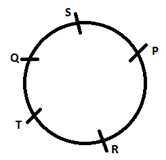Using both the statements it can be found that T sits in between Q and R:

33.What is the position of Peter with respect to Charlie?

I.    Peter stands to the North of Anne, who is to the west of Alex.

II.   Alex is to the North of Charlie.

Answer: e)

Explanation:          Peter is to the North-East of Charlie can be deduced from both the options.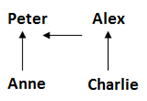34.Village W is in which direction with respect to Village X?

I.    Village W is to the north of Village Y which is to the west of village Z.

II.   Village X is to the west of Village Y.

Answer: e)

Explanation: Village W is to the North-West of Village X can be deduced from both the options.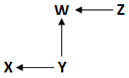35. In a certain code ABC means ‘I got chocolates’. Which alphabet represents chocolates?

I.    XBD means ‘She got sweets’.

II.   APZ means ‘I eat many’.

Answer: e)

Explanation: The options each has a word in common with the question that is ‘got’ and ‘I’, and the letters representing them in the options are common with the alphabets in the question. Hence using both the options it can be concluded that the ‘chocolates’ is represented by C.

36. What is the numerical code for ‘medicine’ in a certain code?

I.    The code for ‘give me medicine’ is ‘819’.

II.   The code for ‘you can bring medicine for me’ is written as ‘584186’.

Answer: d)

Explanation: In I and II, the common words are ‘me’ and ‘medicine’ and the common code numbers are ‘8’ and ‘1’. So, the code for ‘medicine’ is either ‘8’ or ‘1’.

37. How many daughters does X have amongst his children P, Q, R, S and T?

I.    P and Q are sisters of R.

II.   S is brother of Q and T.

Answer: d)

Explanation: The sex of R and T cannot be determined.

38. Who is the father of A?

1. A and B are sisters.
2. B’s mother has three kids.

Answer: d)

Explanation: Both statements I and II together are not sufficient to answer the question.

Directions (39 - 40): An electronic machine rearranges a set of words as illustrated below. Understand the logic behind the rearrangement and answer the question given below:

Input: There is no fight between two friends

Step 1:  fight There is no between two friends

Step 2: no between two friends fight there is

Step 3: friends no between two fight there is

Step 4: two fight there is friends no between

Step 5: is two fight there friends no between

39.       What will be the third step if the input given is ‘what for is the explanation given by’?

a)      Given the what for by is explanation

b)      Explanation is by what the given for

c)       For by what is the given explanation

d)      By is explanation given the what for

e)      None of the above

Answer: d)

40. What will be the fifth step if the input given is ‘what for is the explanation given by’?

a)      For by is explanation given the what by is reason

b)      By is explanation for the given what

c)       For given the what by explanation is

d)      For given the what is by explanation

e)      None of the above

Answer: a)

Banking Awareness Quiz for Upcoming Bank exams

Comment ()

9 + 2 =
Post